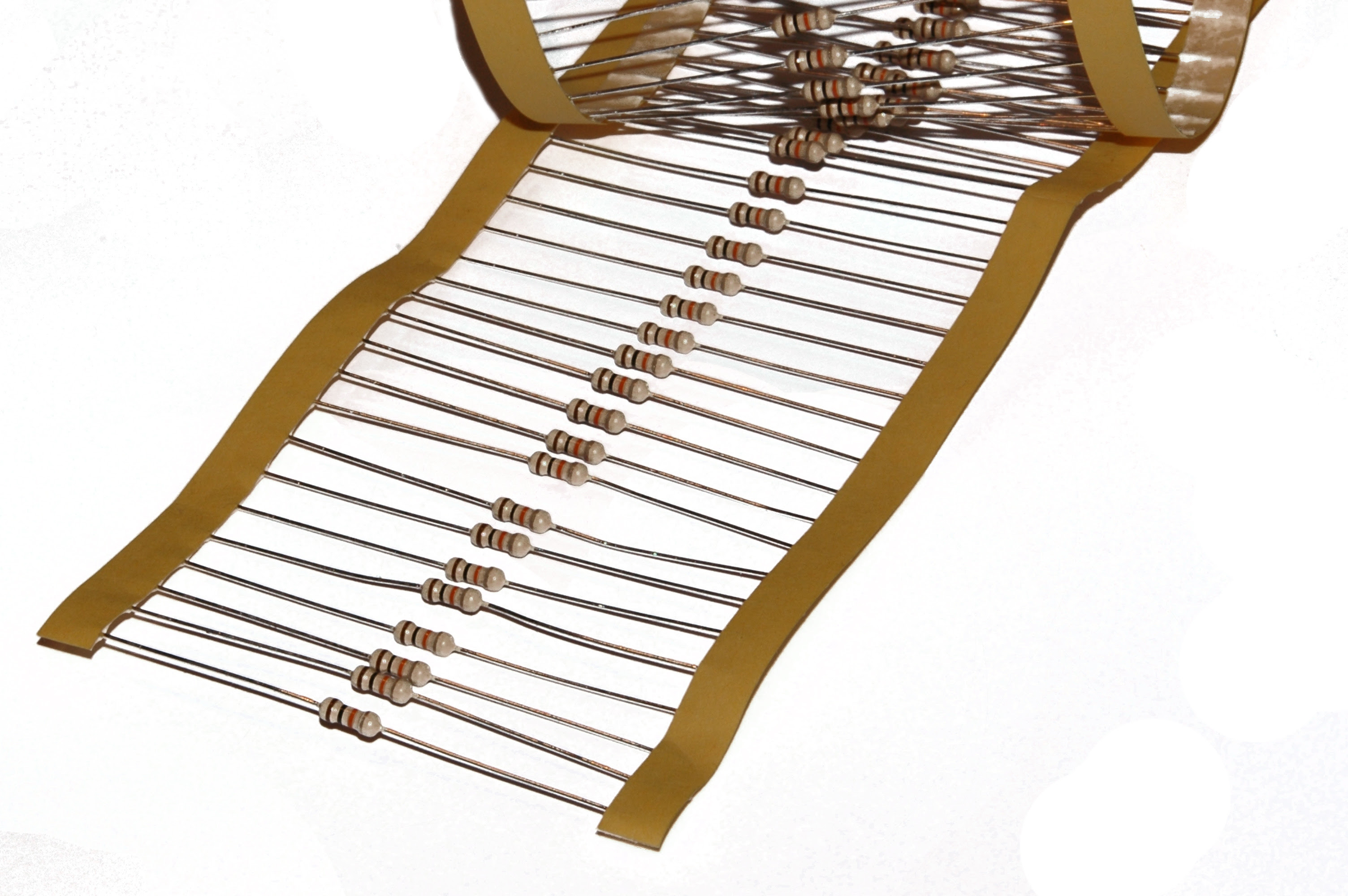### FCC Technician Exam Question Of The Day (T6A01)

Q) What electrical component is used to oppose the flow of current in a DC circuit?

A) Resistor

NJ2X Notes:
These are axial lead resistors on a tape dispenser:Resistors

Ohms is the unit of measure for resistance.  The current through a resistor is in direct proportion to the voltage across the resistor's terminals.  Ohm's law relates current (I) with voltage (E) and resistance (R):

I = E / R